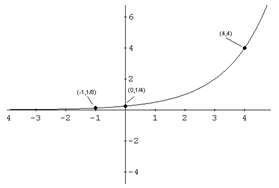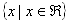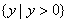From a Graph

To find domain and range of an exponential function by looking at a graph, determine where the x and y-values of the graph are bounded. For domain, look at the graph and find what values of x will be included in the graph. For range, look at the graph and find what values of y are included in the graph.In the graph above, since all x values appear to be present in the graph, the domain is all real numbers or. Since the horizontal asymptote is y = 0, there will not be any values below, or at, y = 0. The range is y > 0, or.

Listen